# Ratios Proportions Lesson 8 1 Ratio a ratio

• Slides: 12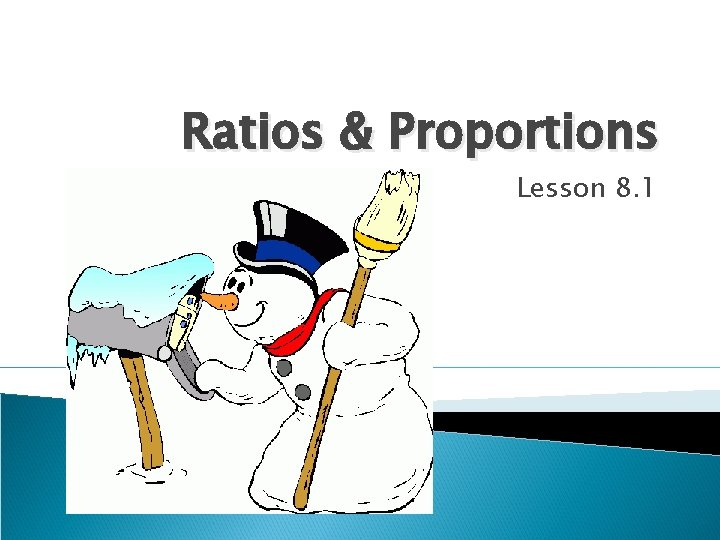Ratios & Proportions Lesson 8. 1Ratio: a ratio is a quotient of two numbers. a: b a to b Always given in lowest terms. Slope of a line is a ratio between two points. (rise over run) a÷bProportions: two or more ratios set equal to each other. = a: b = c: d a is the first term b is the second term c is the third term d is the fourth termProduct and Ratio Theorems In a product containing four terms: First and fourth terms are the extremes. Second and third terms are the means. Theorem 59: In a proportion, the product of the means is equal to the product of the extremes. (means-extremes product theorem. )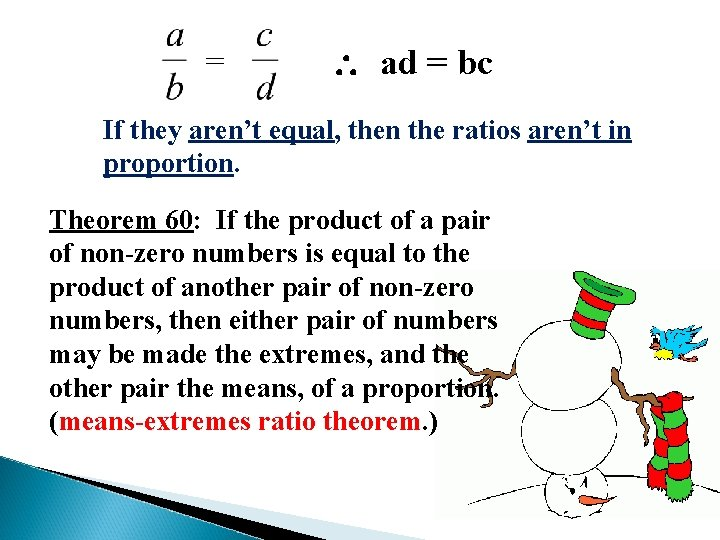= ad = bc If they aren’t equal, then the ratios aren’t in proportion. Theorem 60: If the product of a pair of non-zero numbers is equal to the product of another pair of non-zero numbers, then either pair of numbers may be made the extremes, and the other pair the means, of a proportion. (means-extremes ratio theorem. )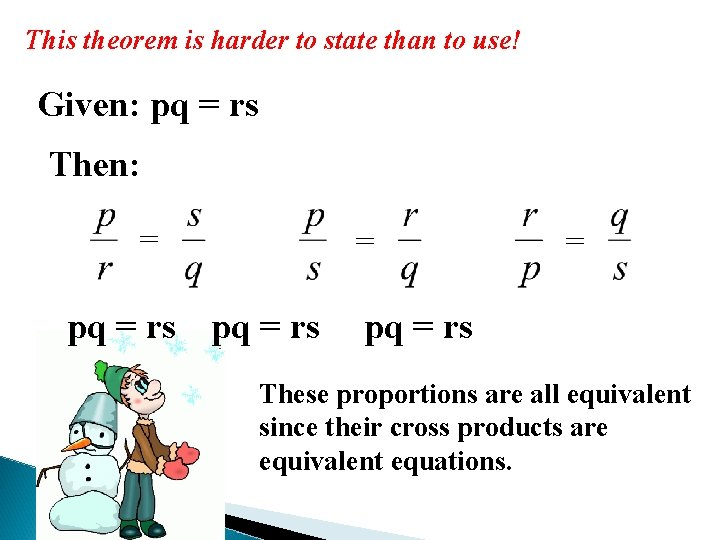This theorem is harder to state than to use! Given: pq = rs Then: = = pq = rs These proportions are all equivalent since their cross products are equivalent equations.In a mean proportion, the means are the same. = 4 is the geometric mean = x is the geometric mean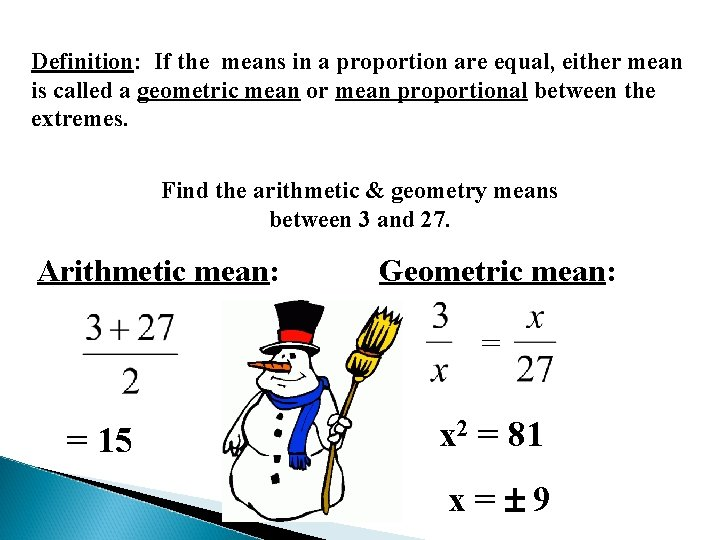Definition: If the means in a proportion are equal, either mean is called a geometric mean or mean proportional between the extremes. Find the arithmetic & geometry means between 3 and 27. Arithmetic mean: Geometric mean: = = 15 x 2 = 81 x= 9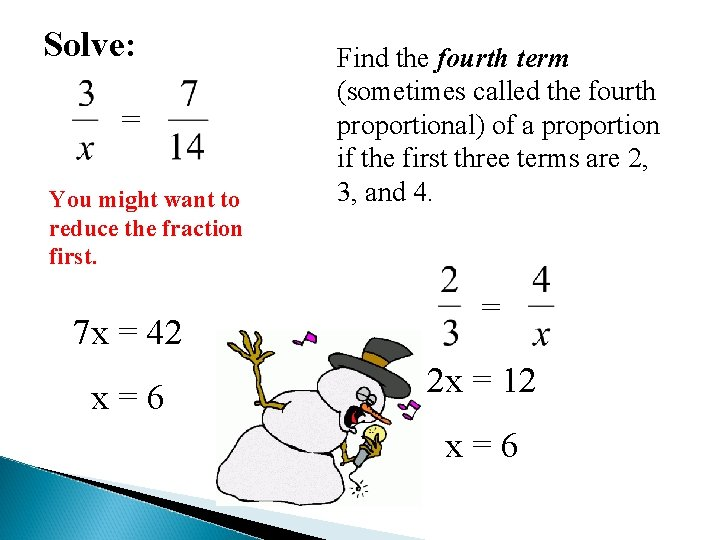Solve: = You might want to reduce the fraction first. 7 x = 42 x=6 Find the fourth term (sometimes called the fourth proportional) of a proportion if the first three terms are 2, 3, and 4. = 2 x = 12 x=6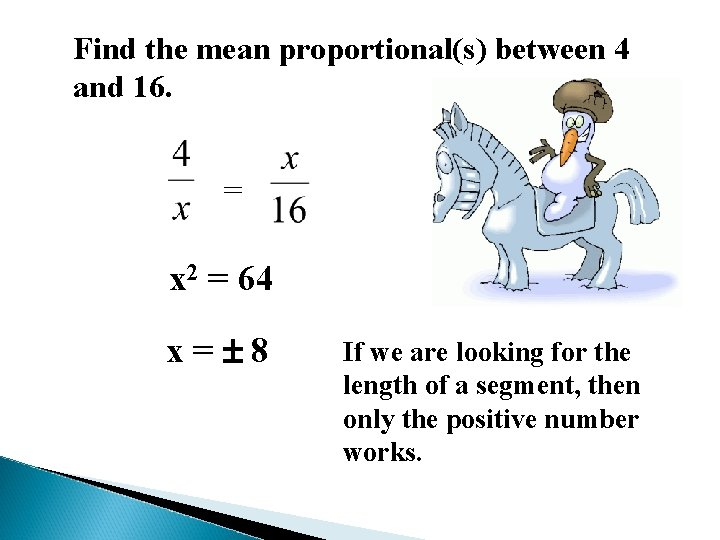Find the mean proportional(s) between 4 and 16. = x 2 = 64 x= 8 If we are looking for the length of a segment, then only the positive number works.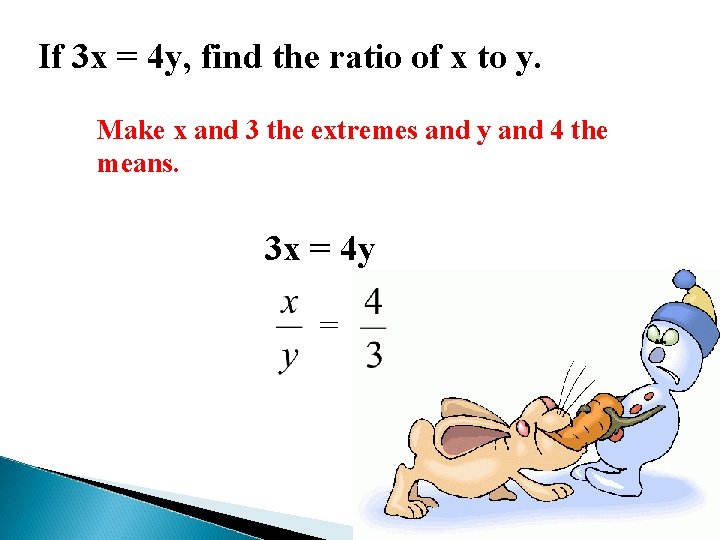If 3 x = 4 y, find the ratio of x to y. Make x and 3 the extremes and y and 4 the means. 3 x = 4 y =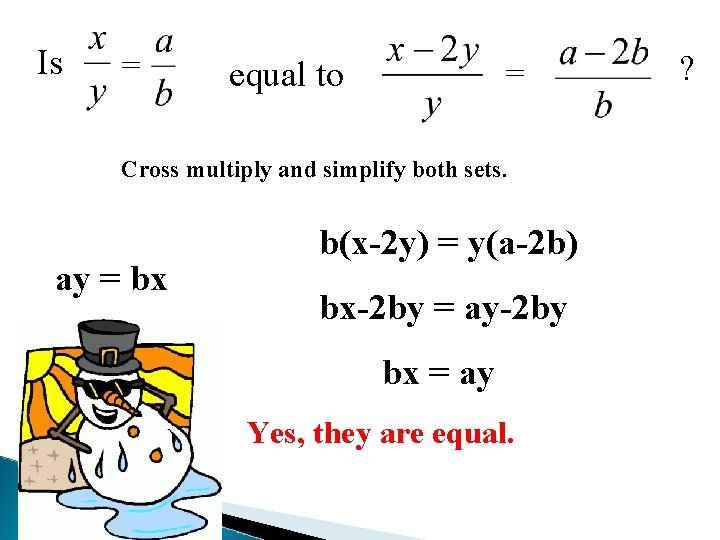Is = equal to = Cross multiply and simplify both sets. ay = bx b(x-2 y) = y(a-2 b) bx-2 by = ay-2 by bx = ay Yes, they are equal. ?